# Ex 13.7 NCERT Solutions- Surface Areas and Volumes Class 9 Notes | EduRev

## Class 9 : Ex 13.7 NCERT Solutions- Surface Areas and Volumes Class 9 Notes | EduRev

The document Ex 13.7 NCERT Solutions- Surface Areas and Volumes Class 9 Notes | EduRev is a part of the Class 9 Course Mathematics (Maths) Class 9.
All you need of Class 9 at this link: Class 9

Note: Assume π = (22/7), unless stated otherwise.

Question 1. Find the volume of the right circular cone with (i) radius 6 cm, height 7 cm (ii) radius 3.5 cm, height 12 cm.
Solution:
(i) Here, radius of the cone r = 6 cm Height (h) = 7 cm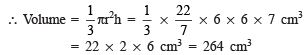(ii) Here, radius of the cone (r) = 3.5 cm = (35/10) cm
Height (h) = 12 cm

∴ Volume of the cone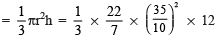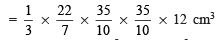= 11 x 7 x 2 cm3 = 154 cm3

Question 2. Find the capacity in litres of a conical vessel with (i) radius 7 cm, slant height 25 cm (ii) height 12 cm, slant height 13 cm
Solution:
(i) Here, r = 7 and l = 25 cm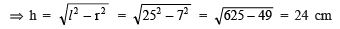∴ Volume of the conical vessel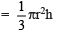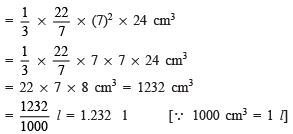Thus, the required capacity of the conical vessel is 1.232 l.

(ii) Here, height (h) = 12 cm and l = 13 cm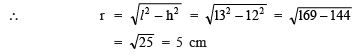Now, volume of the conical vessel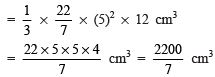∴ Capacity of the conical vessel =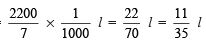Thus, the required capacity of the conical vessel is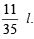Question 3. The height of a cone is 15 cm. If its volume is 1570 cm3, find the radius of the base. (Use π = 3.14)
Solution:
Here, height of the cone (h) = 15 cm
Volume of the cone (v) = 1570 cm
Let the radius of the base be ‘r’ cm.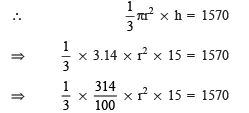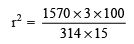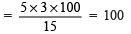⇒ r2 = 102
⇒ r = 10 cm
Thus, the required radius of the base is 10 cm.

Question 4. If the volume of a right circular cone of height 9 cm is 48p cm3, find the diameter of its base.
Solution:
Volume of the cone = 48 p cm3
Height of the cone (h) = 9 cm
Let ‘r’ be its base radius.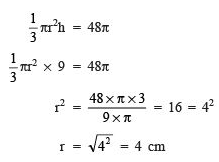∵ Diameter = 2 x Radius
∴ Diameter of the base of the cone = 2 x 4 = 8 cm

Question 5. A conical pit of top diameter 3.5 m is 12 m deep. What is its capacity in kilolitres?
Solution:
Here, diameter of the conical pit = 3.5 m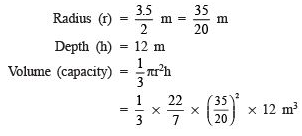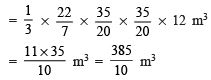= 38.5 m3
∴ 1000 cm3 = 1 l and 1000000 cm3 = 1m3
∴ 1000 x 1000 cm3 = 1000 l = 1 kl
Also 1000 x 1000 cm= 1 m3
⇒ 1 m3 = 1 kl
⇒ 38.5 m3 = 38.5 kl
Thus, the capacity of the conical pit is 38.5 kl.

Question 6. The volume of right circular cone is 9856 cm3. If the diameter of the base is 28 cm, find (i) height of the cone (ii) slant height of the cone (iii) curved surface area of the cone.
Solution:
Volume of the cone (v) = 9856 cm3
Diameter of the base = 28 cm
⇒ Radius of the base =(28/2)cm = 14 cm
(i) To find the height Let the height of the cone be ‘h’ cm.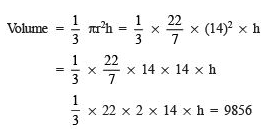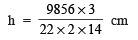= 16 x 3 cm = 48 cm Thus, the required height is 48 cm.

(ii) To find the slant height Let the slant height be ‘ℓ’ cm.
∵ (Slant height)2 = (Radius)2 + (Height)2
∴ ℓ2 = 142 + 482 = 196 + 2304 = 2500 = (50)2
⇒   ℓ = 50
Thus, the required height = 50 cm.

(iii) To find the curved surface area
∵ The curved surface area of a cone is given by πrl
∴ Curved surface area = (22/7) x 14 x 50 cm2
= 22 x 2 x 50 cm
= 2200 cm
Thus, the curved surface area of the cone is 2200 cm2.

Question 7. A right triangle ABC with sides 5 cm, 12 cm and 13 cm is revolved about the side 12 cm. Find the volume of the solid so obtained.
Solution:
Sides of the right triangle are 5 cm, 12 cm and 13 cm.
∵ The right angled triangle is revolved about the 12 cm side.
∴ Its height is 12 cm and base is 5 cm.
Thus, we have Radius of the base of the cone so formed (r) = 5 cm
Height (h) = 12 cm
Slant height = 13 cm
∴ Volume of the cone so obtained  = 1/3πr2h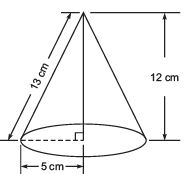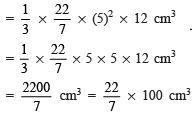= π x 100 cm3                               [∵ π = 22/7]

= 100p cm3
Thus, the required volume of the cone is 100p cm3.

Question 8. If the triangle ABC in the question 7 a bove is revolved about the side 5 cm, then find the volume of the solid so obtained. Find also the ratio of the volumes of the two solids obtained in Questions 7 and 8.
Solution:
Since the right triangle is revolved about the side 5 cm.
∴ Height of the cone so obtained (h) = 5 cm
Radius of the cone (r) = 12 cm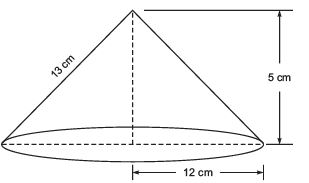∴ Volume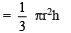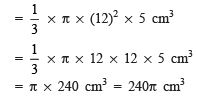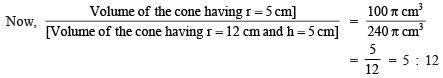Thus, the required ratio is 5 : 12.

Question 9. A heap of wheat is in the form of a cone whose diameter is 10.5 m and height is 3 m.
Find its volume. The heap is to be covered by canvas to πrotect it from rain. Find the area of the canvas required.
Solution:
Here the heap of wheat is in the form of a cone such that Base diameter = 10.5 m
⇒ Base radius (r) = (10.5/2)m = (105/20) m
Height (h) = 3 m Volume of the heap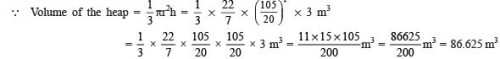Thus, the required volume = 86.625 m
Area of the canvas
∵ The area of the canvas to cover the heap must be equal to the curved surface area of the conical heap.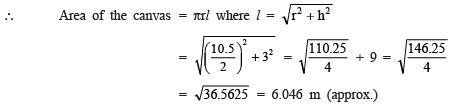= 6.05 m (apπrox.)
Now,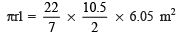= 11 x 1.5 x  6.05 m2
= 99.825 m2

Thus, the required area of the canvas is 99.825 m2.

VOLUME OF A SPHERE

REMEMBER

(i) Volume of a sphere = (4/3) πr3, where ‘r’ is the radius of the sphere.

(ii) Volume of a hemisphere = (2/3)πr3, where ‘r’ is the radius of the hemisphere.

Offer running on EduRev: Apply code STAYHOME200 to get INR 200 off on our premium plan EduRev Infinity!

## Mathematics (Maths) Class 9

190 videos|233 docs|82 tests

,

,

,

,

,

,

,

,

,

,

,

,

,

,

,

,

,

,

,

,

,

;